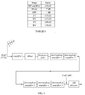# Electronic Gain in Amplifiers

• cjm181
In summary, the conversation discusses the block diagram of a superheterodyne radio receiver, where a 20 μV signal is fed through several stages to eventually reach the input of the AM detector. The AM detector needs a minimum signal level of -3dB(mW) to work properly. The required minimum gain of the fifth intermediate amplifier is calculated using data from Table 1, and is found to be 17.73dB. The use of a spreadsheet is suggested to help balance the energy account.

## Homework Statement

In a test a 20 μV signal was fed from the aerial into the first stage of the
several stages of the receiver to eventually appear at the input to the AM
detector. For the AM detector to work satisfactory it requires a minimum
signal level of –3dB(mW). Calculate the required minimum gain of the
fifth intermediate amplifier given the data in TABLE 1.

Fig 1 looks like the below

Ariel (20microV, 75ohms) > Amplifier > Mixer . Band Pass Filter > Intermetiate Amp 1 > Inter Amp 2 > Inter Amp 3 > Inter Amp 4 > Inter Amp 5 > AM Detector (needs -3dB (mW).

Table 1 is below

RF amp = +10dB
Mixer = -7dB
Band pass filter = -1dB
Intermediate amp = +15dB
IA2 = +15dB
IA3 = +20dB
IA4 = 10dB

## The Attempt at a Solution

Find input power level coming into the system:

P = V^2 / R = 0.00002^2 / 75 = 5.3333e-12W (or 5.3333e-9 mW)

Convert mW to dB(mW)

10 x Log (5.3333e-9) = -82.73 dB(mW)

So, the total system gain is the sum of all gains, so if we have -82.73 in, and want -3 out, then:

-3 = (-82.73) + 10 + (-7) + (-1) + 15 + 15 + 10 + IA5

-3 = -20.73 + IA5

IA5 = -3 + 20.73 = 17.73.

Therefore the gain at intermediate amplifier 5 is 17.73dB (or 18dB)

Is this going in the right direction? or correct?

Thanks

Last edited:
Fig 1 and Table 1

#### Attachments

•Fig 1.jpg
23.4 KB · Views: 352
Input signal is 20uV in 75 ohms. Convert to power, to mW then to dBm.
W = V^2 / R = 20e-6 * 20e-6 / 75 = 5.333e-12W = 5.333e-9 mW
dBm = 10 * Log( 5.333e-9 ) = -82.73 dBm
Code:
Description   Debit   Credit dB    Balance dBm
Input signal   –      -82.73 dBm   -82.73
RF amp         –       10 dB       -72.73
Mixer         7 dB      –          -79.73
BPF           1 dB      –          -80.73
IF Amp 1       –       15 dB       -65.73
IA2            –       15 dB       -50.73
IA3            –       20 dB       -30.73
IA4            –       10 dB       -20.73
Detector       –        3 dBm      -17.73
So; IF amp 5 will need gain a gain of +17.73 dB to balance the energy account.

You are correct.

Thankyou Baluncore.

I didnt think of using a spread sheet. Thanks for the tip...

Kr
Craig